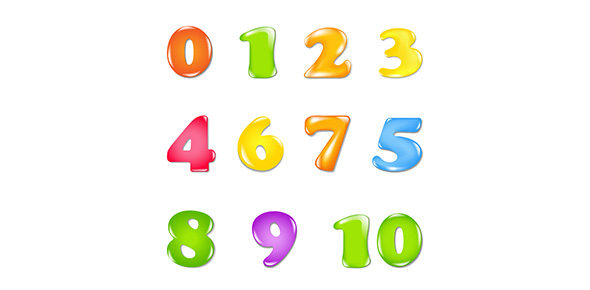# Subtraction Of Whole Numbers

10 Questions | Total Attempts: 260SettingsThis quiz will test your foundation skills in subtraction. If you pass it without the use of a calculator, then you're off to a good start.

• 1.
Which of the following symbols represents subtraction?
• A.

+

• B.

-

• C.

X

• D.

÷

• 2.
What are some other words used to describe a subtraction operation?(Click ALL that apply)
• A.

Minus

• B.

Total

• C.

Decrease

• D.

Icrease

• E.

Take away

• 3.
9 - 6 = 4True or False?
• A.

True

• B.

False

• 4.
What is the first step to solving a subtraction problem?
• A.

Panic

• B.

Get a calcuator

• C.

Rewrite the problem all lined up in columns from the right to the left.

• 5.
Solve this subtraction question using a pencil and paper:(no calculators allowed)41 - 5 =
• 6.
Solve this subtraction question using a pencil and paper:(no calculators allowed)725 - 693 =
• 7.
Solve this subtraction question using a pencil and paper:(no calculators allowed)8536 - 956 =
• 8.
Solve this subtraction question using a pencil and paper:(no calculators allowed)12403 - 1943 =
• 9.
Solve this subtraction question using a pencil and paper:(no calculators allowed)144031 - 85252 =
• 10.
To subtract means to remove a number of items from any quantity and finding out how many items are left over. True or False?
• A.

True

• B.

False

Related TopicsBack to top